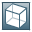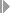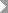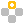Show TOC

###Depreciation Types - Setup Window: General TabUse this tab to define the general parameters for depreciation types.

To access this tab, from the SAP Business One Main Menu, chooseAdministrationSetupFinancialsFixed AssetsDepreciation Typesand select the General tab.

##### General Tab Fields
 Specify the minimum book value of an asset after depreciation.NoteIf you have specified a minimum depreciated value and a salvage value percentage at the same time, the salvage value has higher priority. That is, the system depreciates an asset till the asset value is reduced to the salvage value. End of the note.NoteThe field is available only for depreciation types having the Straight Line or Declining Balance method. End of the note. Enter the maximum depreciable value of an asset. The system takes the maximum depreciable value into account when calculating the depreciation charges for an asset. For more information, see the examples below.ExampleAsset XAcquisition and Production Costs: 80,000 USD Useful Life: 60 Months Maximum Depreciable Value: 60,000 USD Salvage Value Percentage: None Depreciation Method: Straight Line Calculation Method: Acquisition Value / Total Useful Life Calculation Base: Monthly As the maximum depreciable value is 60,000 USD, the system calculates the monthly depreciation as follows: 60000 USD / 60 = 1000 USD At the end of the useful life, the asset still holds the value of 20,000 USD. Asset YAcquisition and Production Costs: 30,000 USD Useful Life: 60 Months Maximum Depreciable Value: 60,000 USD Salvage Value Percentage: None Depreciation Method: Straight Line Calculation Method: Acquisition Value / Total Useful Life Calculation Base: Monthly As the acquisition and production costs are less than the maximum depreciable value, the asset can be depreciated until its acquisition and production costs reach zero. Therefore, the monthly depreciation charge is calculated as follows: 30000 USD / 60 = 500 USD At the end of the useful life, the asset's book value is zero. Asset ZAcquisition and Production Costs: 15,000 USD Useful Life: 60 Months Maximum Depreciable Value: 9,000 USD Salvage Value Percentage: 20% Depreciation Method: Straight Line Calculation Method: Acquisition Value / Total Useful Life Calculation Base: Monthly The system calculates the salvage value of the asset as follows: 15000 USD * 20% = 3000 USD Though the asset's maximum depreciable value is 9,000 USD, its actual depreciable value is only 6000 USD, considering the salvage value. Therefore, the asset's monthly depreciation charge is calculated as follows: Include Salvage Value in Depreciation Calculation checkbox: Selected9000 USD / 60 = 150 USD After 40 months, the asset's accumulated depreciation charges reach 6,000 USD and the depreciation stops. Include Salvage Value in Depreciation Calculation checkbox: Unselected(9000 USD – 3000 USD) / 60 = 100 USD End of the example.
 Rounds the net book values of assets at the end of each fiscal year. Once you select the checkbox, SAP Business One rounds the depreciation amounts of the fiscal year so that the year end book values of the assets are rounded.ExampleThe net book value of an asset calculated by the system amounts to 28,123.32 USD at the end of the year. If you have selected the checkbox, the system rounds this amount to 28,123.00 USD. The depreciation amount of the asset in the year is therefore increased by 0.32 USD. End of the example.
 Enter a salvage value percentage.NoteIf you have specified a minimum depreciated value and a salvage value percentage at the same time, the salvage value has higher priority. That is, the system depreciates an asset till the asset value is reduced to the salvage value. End of the note.
 Includes the salvage value in the calculation of an asset's depreciation.ExampleAsset X Acquisition and Production Costs: 6,000 USD Useful Life: 60 Months Depreciation Method: Straight Line Calculation Base: Yearly Calculation Method: Acquisition Value / Total Useful Life Salvage Value Percentage: 10% If you have not selected the checkbox, the system calculates the asset's monthly depreciation as follows:(6000 USD – 6000 USD * 10%) / 60 = 90 USD In this case, the asset is depreciated by 90 USD per month, and the salvage value is 600 USD after its 60 months useful life. If you have selected the checkbox, the system calculates the asset's monthly depreciation as follows:6000 USD / 60 = 100 USD In this case, the asset is depreciated by 100 USD per month in the first 4 years, and 50 USD per month in the last year of its useful life so that its salvage value remains 600 USD. End of the example.
 Specify how the acquisition of an asset determines the asset's depreciation start date. From the dropdown list, select one of the following conventions: Pro Rata Temporis – If you select this, the PR Temporis Type field appears. From the dropdown list, you can select one of the following options to determine the depreciation start date: Exact Daily Base – The depreciation of the asset starts from the day of the asset acquisition.First Day of Current Period – The depreciation of the asset starts from the first day of the posting period within which the asset acquisition takes place.First Day of Next Period – The depreciation of the asset starts from the first day of the posting period that follows the period of asset acquisition. First Year Convention – Determines the depreciation start date as follows: When your fiscal year matches a calendar year: If the asset acquisition takes place before July 1st, the depreciation starts from January 1st.If the asset acquisition takes place after July 1st, the depreciation starts from July 1st. When your fiscal year does not match a calendar year: If the asset acquisition takes place in the first half of the fiscal year, the depreciation starts from the first day of the first half of the year.If the asset acquisition takes place in the second half of the fiscal year, the depreciation starts from the first day of the second half of the year. Half Year – The depreciation of the asset always starts from the first day of the second half of the fiscal year during which the asset acquisition takes place.Full Year – The depreciation of the asset always starts from the first day of the fiscal year during which the asset acquisition takes place.
 Specify how an asset's subsequent acquisition affects the asset's depreciation. From the dropdown list, select one of the following conventions: Pro Rata Temporis – If you select this, the field PR Temporis Type appears. From the dropdown list, you can select one of the following options: Exact Daily Base – Recalculates the asset depreciation from the very day when the subsequent acquisition takes place.First Day of Current Period – Recalculates the asset depreciation from the first day of the posting period within which the subsequent acquisition takes place.First Day of Next Period – Recalculates the asset depreciation from the first day of the posting period that follows the period of subsequent acquisition. Half Year Convention – Recalculates the asset depreciation as one of the following: If an asset's subsequent acquisition takes place in the fiscal year of acquisition, the system recalculates the asset depreciation from the very day when the depreciation starts.If an asset's subsequent acquisition takes place in years that follow the fiscal year of acquisition, the system recalculates the asset depreciation from the first day of the fiscal year during which the subsequent acquisition occurs. Full Year – Recalculates the asset depreciation as one of the following: If an asset's subsequent acquisition takes place in the fiscal year of acquisition, the system recalculates the asset depreciation from the very day when the depreciation starts.If an asset's subsequent acquisition takes place in years that follow the fiscal year of acquisition, the system recalculates the asset depreciation from the first day of the fiscal year during which the subsequent acquisition occurs.
 Specify how an asset's retirement affects the asset's depreciation. From the dropdown list, select one of the following conventions: Pro Rata Temporis – If you select this, the field PR Temporis Type appears. From the dropdown list, you can select one of the following options: Exact Daily Base – Calculates the asset depreciation till the very day of retirement.Last Day of Prior Period – Calculates the asset depreciation till the last day of the posting period prior to the period of retirement.Last Day of Current Period – Calculates the asset depreciation till the last day of the posting period in which the retirement takes place. Half Year Convention – Calculates the asset depreciation as one of the following: If the asset retirement takes place in the first half of the fiscal year, the system calculates the depreciation till the last day of the first half of the year.If the asset retirement takes place in the second half of the fiscal year, the system calculates the depreciation till the last day of the second half of the year. Only After the End of Useful Life – Select this for low value assets, which can be retired only after the end of their useful life. Note that this option is available in the Germany localization only.
Include Depreciation of Previous Periods in Capitalization Period

Moves depreciation of previous periods in the fiscal year to the capitalization period.

In Italy, for instance, in the year of asset capitalization, it is legally required to calculate the asset depreciation for the entire year and the deprecation start date cannot be earlier than the capitalization date.

If the capitalization of an asset does not take place at the beginning of a year, you can calculate the depreciation for the entire year and then select the checkbox to move the depreciation of the previous periods to the capitalization period.Example

Asset X

Acquisition and Production Costs: 10,000 USD

Useful Life: 60 Months

Depreciation Method: Straight Line

Calculation Method: Acquisition Value / Total Useful Life

Capitalization Date: April 1st, 2012

It is required to deduct the depreciation in the first year by 50%.

The system first calculates the depreciation in the first year as follows:

(10000 USD / 60 * 12) * 50% = 1000 USD

Then, the depreciation per month is calculated as follows:

1000 USD / 12 = 83.33 USD

• If you have selected this checkbox, the asset depreciation in 2012 is as follows:

Jan.

Feb.

Mar.

Apr.

May

Jun.

Jul.

Aug.

Sep.

Oct.

Nov.

Dec.

333.32

83.33

83.33

83.33

83.33

83.33

83.33

83.33

83.33

• If you have not selected this checkbox, the asset depreciation in 2012 is as follows:

Jan.

Feb.

Mar.

Apr.

May

Jun.

Jul.

Aug.

Sep.

Oct.

Nov.

Dec.

83.33

83.33

83.33

83.33

83.33

83.33

83.33

83.33

83.33

83.33

83.33

83.33

End of the example.
Stop Depreciation at End of Last Full Fiscal Year

Stops an asset's depreciation at the end of the last full fiscal year of the asset's useful life.Example

Asset X

Acquisition and Production Costs: 3,600 USD

Useful Life: 36 Months

Depreciation Method: Straight Line

Calculation Base: Monthly

Calculation Method: Acquisition Value / Total Useful Life

Capitalization Date: April 1st, 2010

• If you have selected this checkbox, the asset depreciation is as follows:

Jan.

Feb.

Mar.

Apr.

May

Jun.

Jul.

Aug.

Sep.

Oct.

Nov.

Dec.

2010

100

100

100

100

100

100

100

100

100

2011

100

100

100

100

100

100

100

100

100

100

100

100

2012

100

100

100

100

100

100

100

100

100

100

100

400

• If you have not selected this checkbox, the asset depreciation is as follows:

Jan.

Feb.

Mar.

Apr.

May

Jun.

Jul.

Aug.

Sep.

Oct.

Nov.

Dec.

2010

100

100

100

100

100

100

100

100

100

2011

100

100

100

100

100

100

100

100

100

100

100

100

2012

100

100

100

100

100

100

100

100

100

100

100

100

2013

100

100

100

End of the example.
Percentage of Depreciation to Reverse in Retirement Year

Enter the amount (in percentage) of depreciation you want to reverse for an asset in the retirement year.

After you specify the percentage, the system decreases the asset's planned depreciation in the retirement year, and adjusts its net book value accordingly.Note

The percentage you specify here applies to an asset only when it is fully retired.

End of the note.Example

Asset X

Acquisition and Production Costs: 48,000 USD

Useful Life: 48 Months

Depreciation Method: Straight Line

Calculation Method: Acquisition Value / Total Useful Life

Percentage of Depreciation to Reverse in Retirement Year: 40%

Capitalization Date: January 1st, 2010

Retirement Date: July 1st, 2012

The system calculates the asset depreciation as follows:

Jan.

Feb.

Mar.

Apr.

May

Jun.

Jul.

Aug.

Sep.

Oct.

Nov.

Dec.

2010

1000

1000

1000

1000

1000

1000

1000

1000

1000

1000

1000

1000

2011

1000

1000

1000

1000

1000

1000

1000

1000

1000

1000

1000

1000

2012

1000

1000

1000

1000

1000

1000

2013

Then, the system calculates the depreciation to reverse in 2012 as follows:

1000 USD * 6 * 40% = 2400 USD

And the deprecation in 2012 is updated as follows:

(6000 USD – 2400 USD) / 6 = 600 USD

Jan.

Feb.

Mar.

Apr.

May

Jun.

Jul.

Aug.

Sep.

Oct.

Nov.

Dec.

2012

600

600

600

600

600

600

The net book value of the asset on July 1st, 2012 is 20,400 USD.

End of the example.
 Specify the valid period of the depreciation type.